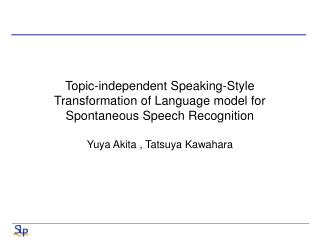DownloadDownload PresentationYuya Akita , Tatsuya Kawahara

Yuya Akita , Tatsuya Kawahara

Télécharger la présentationYuya Akita , Tatsuya Kawahara

- - - - - - - - - - - - - - - - - - - - - - - - - - - E N D - - - - - - - - - - - - - - - - - - - - - - - - - - -
Presentation Transcript

1. Topic-independent Speaking-Style Transformation of Language model for Spontaneous Speech Recognition Yuya Akita , Tatsuya Kawahara

2. Introduction • Spoken-style v.s. writing style • Combination of document and spontaneous corpus • Irrelevant linguistic expression • Model transformation • Simulated spoken-style text by randomly inserting fillers • Weighted finite-state transducer framework (?) • Statistical machine translation framework • Problem with Model transformation methods • Small corpus, data sparseness • One of solutions: • POS tag

3. Statistical Transformation of Language model • Posteriori: • X: source language model (document style) • Y: target language model (spoken language) • So, • P(X|Y) and P(Y|X) are transformation model • Transformation models can be estimated using parallel corpus • n-gram count:

4. Statistical Transformation of Language model (cont.) • Data sparseness problem for parallel corpus • POS information • Linear interpolation • Maximum entropy

5. Training • Use aligned corpus • Word-based transformation probability • POS-based transformation probability • Pword(x|y) and PPOS(x|y) are estimated accordingly

6. Training (cont.) • Back-off scheme • Linear interpolation scheme • Maximum entropy scheme • ME model is applied to every n-gram entry of document-style model • spoken-style n-garm is generated if transform probability is larger than a threshold

7. Experiments • Training coprus: • Baseline corpus: National Congress of Japan, 71M words • Parallel corpus: budget committee in 2003, 666K • Corpus of Spontaneous Japan, 2.9M words • Test corpus: • Another meeting of Budget committee in 2003, 63k words

8. Experiments (cont.) • Evaluation of Generality of transformation model • LM

9. Conclusions • Propose a novel statistical transformation model approach

10. Non-stationary n-gram model

11. Concept • Probability of sentence • n-gram LM • Actually, • Miss long-distance and word position information while applying Markov assumption

12. Concept (cont.)

13. Training (cont.) • ML estimation • Smoothing • Use low order • Use small bins • Transform with Smoothed normal ngram • Combination • Linear interpolation • Back-off

14. Smoothing with lower order (cont.) • Additive smoothing • Back-off smoothing • Linear interpolation

15. Smoothing with small bins (k=1) (cont.) • Back-off smoothing • Linear interpolation • Hybrid smoothing

16. Transformation with smoothed ngram • Novel method • If t-mean(w) decreases, the word is more important • Var(w) is used to balance t-mean(w) for active words • active word: words can appears at any position in the sentences • Back-off smoothing & linear interpolation

17. Experiments Observation: Marginal position & middle position

18. Experiments (cont.) • NS bigram

19. Experiments (cont.) • Comparison with three smoothing techniques

20. Experiments (cont.) • Error rate with different bins

21. Conclusions • Traditional n-gram model is enhanced by relaxing its stationary hypothesis and exploring the word positional information in language modeling

22. Two-way Poisson Mixture model

23. Document x Class k X1 X2 Poisson 1 ... πk1 xp πk2 Poisson 2 Σ … πkRk Poisson Rk Essential • Poisson distribution • Poisson mixture model Multivariate Poisson, dim = p (lexicon size) *Word clustering: reduce Poisson dimension => Two-way mixtures